# In a typical Machine Learning project, one would need to find out how good or bad their models are by measuring the models’ performance on a test dataset, using some statistical metrics.

Various performance metrics are used for different problems, depending on what needs to be optimized by the models. For this blog, we will focus on the evaluation metrics that are used in weather forecasting, based on radar images.

The major problem that we need to overcome in our forecasting model is to quickly, precisely, and accurately predict the movement of rain clouds in a short period. If heavy rain is predicted as showers or – even worse – as cloudy weather without rain, the consequences could be serious for the users of our prediction model. If the rain is going to stop in the next few minutes, incorrect forecasting – that predicts that rainfall would continue with high intensity – may cause little to no harm; however, the prediction model is no longer useful.

A good model should tackle as many of these issues as possible. We believe that the following measures may help us identify which model is better.

# Performance Metrics

• Root Mean Square Error (RMSE)This is a broad measure of accuracy in terms of an average error across the value of forecast-observation pairs. Formally, it is defined as follows: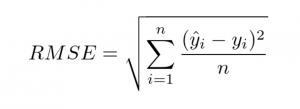This measure will help us to compare how much difference intensity between ground truth observation and predicted one.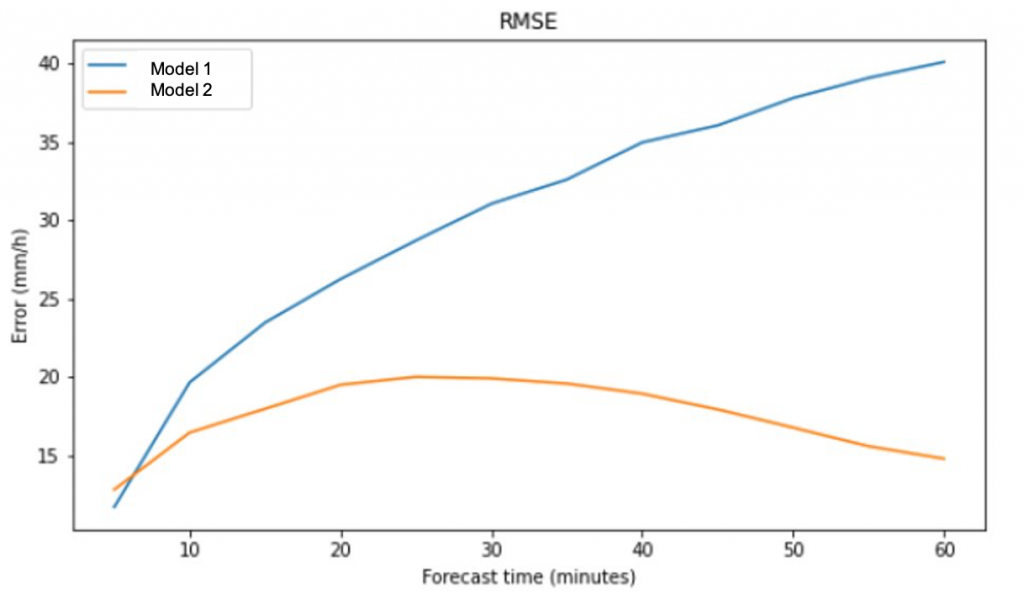Figure 1: RMSE between 2 models across 60 minutes forecast.

Figure 1 shows an example of the RMSE between 2 different models over 60 minutes forecast.

The RMSE  of Model 1  is increasing over time. On the other hand, it seems that Model 2 has a smaller RMSE, which means it is a better model out of the two models.

Before defining the next metric, we need to recall about Confusion Matrix (Figure 2). Each column of the matrix represents the instances in an actual class while each row represents the instances in a predicted class, or vice versa . By using Confusion Matrix, we can calculate the number of False Positives (FP), False Negatives (FN), True Positives (TP), and True Negatives (TN).

• Hit Rate (H): The fraction of observed events that are forecast correctly. This is also known as the Probability of Detection.​ It tells us what proportion had rain was predicted by the algorithm as having rain. It ranges from [0,1].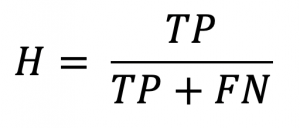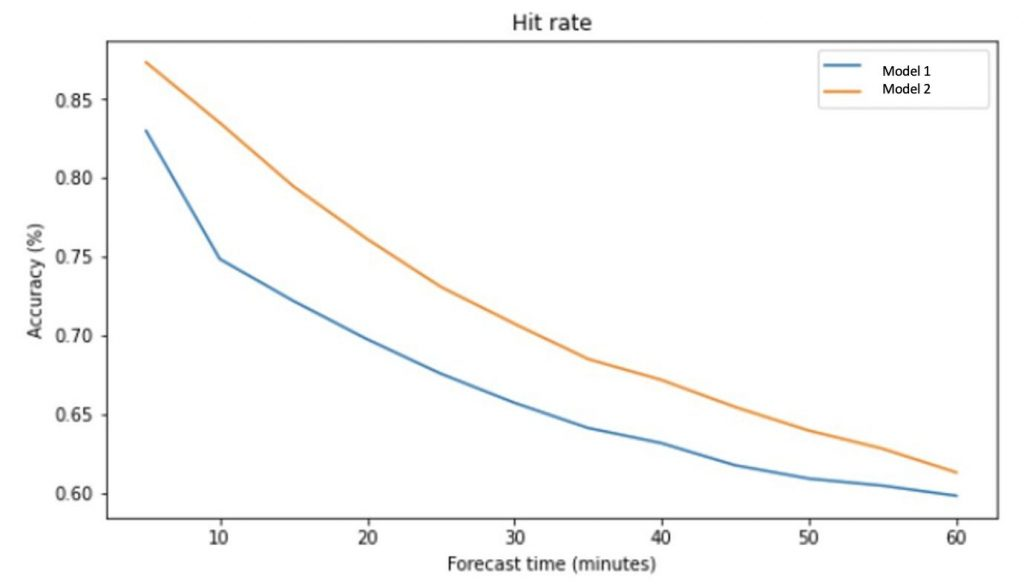Figure 3: Hit rate of 2 models across 60 forecast times.

From Figure 3, the Hit Rate of both models is good in the first 20 minutes. Model 2 has a higher value than model 1 (higher probability of predicting rain). Therefore,  model 2 is the better model base on this measure.

• False Alarm Ratio (FAR): The fraction of “yes” forecasts that were wrong. It is calculated as follows: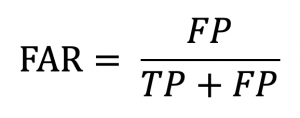Even though in weather forecast the False Alarms do not lead to serious consequences. However,  a model with a high FAR measure is not ideal.

• Bias (B): This measure compares the number of points is predicted as having rain and the total number of actual rain points.  Specifically,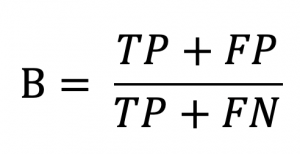## Radar Image Compressing by Huffman Algorithm

In AI Lab, one of our projects is “Weather Forecasting”, in which we process the data from weather radars, and perform prediction using nowcasting techniques, i.e.  forecasting in a short period like 60 minutes.

To develop a forecasting model and do an evaluation, we need to run many test cases. Each test case corresponds to one rainfall event, whose duration can be several hours. In the rainy season, one rainfall event cans last for several days.

Given one event and one interesting area (80km2 of Tokyo city for example). In our actual work, a list of arrays of rainfall intensity needs to be stored in a machine. Each array corresponds to one minute of observation by radar. If the event lasts for 5 days, then the number of arrays is

60 minutes x 24 hours x 5 days = 7200 arrays

Therefore, storing such an amount of data costs a lot of memory.

One solution is to modify the way feeding data to the evaluation module. However, for the scope of this blog, let assume that we would like to keep that amount of data on the computer. How do we do to compress data, and how much data can be compressed?

The post aims to introduce the Huffman algorithm, one of the basic methods for data compressing. By learning about the Huffman algorithm, we can easily understand the mechanism behind some advanced compressing methods.

Let us start with a question: how does a computer store data?

# Data in computer language

Computer uses bits of {0,1} to represent numbers. One character of 0 or 1 is called a bit. Any string of bits is called a code.

Each number is encoded by a unique string of bits, or code. The encoder should satisfy the “prefix” condition. This condition states that no code is the prefix of any other codes. This makes it is always possible to decode a bit-string back to a number.

Let us consider an example. Let assume that we have a data d as a list of numbers, d = [1, 1, 1, 2, 2, 2, 3, 3, 3, 3].

To store this list d, the computer needs to encode the three numbers: 1, 2, 3. The two possible encoders are:

 Code/Number 1 2 3 Code 1 ‘0’ ‘01’ ‘11’ Code 2 ‘0’ ‘10’ ‘11’

But the Code 1 violates the ‘prefix’ condition, as ‘0’ is a prefix of ’01’, while Code 2 does not. Therefore, Code 2 can be used for encoding.

So, how many bits needed to represent N different numbers? In theoretically, we need codes of k-bits length to encode 2^k numbers.

For example, to encode 2 numbers, we need codes of 1-bit length, which are ‘0’ and ‘1’.

To encode 4 = 2^2 numbers, we need codes of 2-bits length, which are: ’00’, ’01’, ’10’, ’11’

To encode 256 = 2^8 numbers, we need codes of 8-bits length, which are: … [we skip it :)]

Consider one radar image of dimension 401×401 including float numbers that take value from 0 to 200 mm/h. If we are interested in one decimal number, this data can be multiplied by 10 and saved as an integer. At that time, the array contains 401×401 integers, whose values are from 0 to 2000.

Following the above logic, to encode 2000 different numbers, we need 11-bits codes, because 2^11 = 2048. If so, the total number of bits to encode this data is: 401 x 401 x 11 bits = 1,768,811 bits.

Compressing data aims to reduce the total number of bits that are used to encode it, in other words, to find an encoder that has a minimum average of code lengths.

# Expected code length

The expected or average code length (ECL) of a data d is defined as: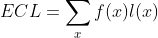where x is the values of data, f(x) is its frequency, and l(x) is the length of code used to encode the value x. This quantity tells us how many bits on average are used to encode a number in the data x and by a Code C.

# Minimum expected code length

It can be proved mathematically that, the ECL of a code is bounded below by the entropy of given data. What is the entropy of data? It’s a quantity to measure information, that was initially introduced by Shannon (a Mathematician and father of Information Theory). This quantity tells us how much uncertainty the data contains.

From the mathematical point of view, the entropy of the data is defined as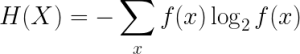And by the source coding theorem, the following inequality is always true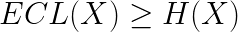which is equivalent to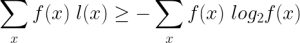The ECL achieves the minimum value if l(x) = -log2 [f(x)], meaning that, the length of the code of value x depends on the frequency of that value in the data. For example, in our radar images, the most frequent number is 0 (0.1mm/h), then we should use a short string of bits to encode the value 0. On the contrary, the number 2000 (0.1mm/h) has a very low frequency, then a longer bit-string must be used. In that way, the average of bits used to encode one value will be reduced to the entropy value, which is the lower bound of the compression. If we use a code that yields an ECL less than the entropy, then the compression makes a loss of information.

In summary, the compressing could be minimized if the length of codes follows the formula: l(x) = -log2 [f(x)].

# The Huffman Encoding

The Huffman algorithm is one of the methods to encode data into bit-strings whose ECL approximates the lower bound of compression. This is a very simple algorithm that can be produced by several code lines in Python. For details of the Huffman algorithm, you can refer to the link:

https://www.geeksforgeeks.org/huffman-coding-greedy-algo-3/

# Encode and Decode radar images

As described above, a radar image contains 401×401 data points, each point is an integer taking value from 0 to 2000.

By performing the Huffman encoder, we obtained the following codes (Figure 1).

By using these codes, the total number of bits of one specific image is 416,924 bits, which is much smaller than the codes using 11-bits length.

In actual work, we can convert image data to bits by concatenate value by value, then write everything to a binary file. As the computer groups every 8 bits to a byte, then the binary file consumes 416,924/8 bits = 52KB.

To decode a binary file back to the image array, we load the binary file and transform it to bit. As the Huffman code verifies the ‘prefix’ condition, by searching and mapping, the original data can always be reconstructed without losing any information.

If we write the image array in the numpy format, then the storage can be up to 1.3MB/array. Now with Huffman compression, the storage can be reduced to 52KB.

If we use the ‘ZIP’ application to compress the numpy file, the storage of the zip file is only 48KB, meaning that the ‘ZIP’ is outperforming our Huffman encoding. That is because ZIP is using another encoding algorithm that allows reaching closer to the lower bound of compression.

# Hiring Data Scientist / Engineer

We are looking for Data Scientist and Engineer.